IMPORTANT: RRB EXAM PORTAL is NOT associated with Railway Recruitment Board(RRB) or Indian Railways.

# (Paper) (RPF) Exam paper held on 10 January 2019 (Reasoning)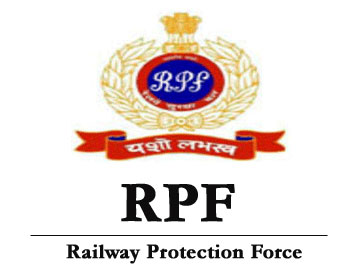## (Paper) (RPF) Exam 2019 held on 10 January 2019 Reasoning Set-1 (English)

Instructions

For the following questions answer them individually

Question 51. How many straight lines are there in the given figure?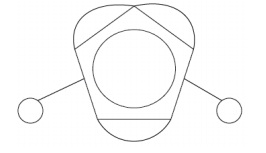A    11

B    8

C    7

D    10

Question 52. Which one of the alternative will be the next figure from the given alternatives for the given series?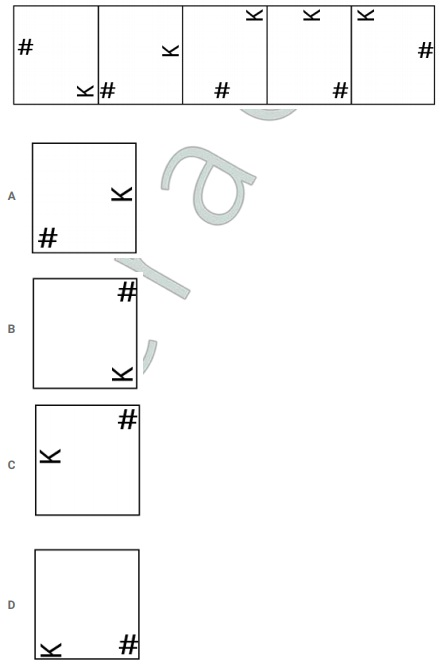Question 53. Replace the question mark with an option that follows the same logic applied in the first pair X:L::M: ??

A     A

B    C

C    V

D    S

Explanation:

In the relation X:L::M: ??,

X Position as per alphabetical order is 24 and L position is 12. Difference in value of their positions will be 12.

M Position is 13. To get difference of 12 for M, It should be letter in Position 1 which is A. Hence, Option A is right choice.

Question 54. Replace the question mark with an option that follows the same logic applied in the first pair Net:Fish::Antenna: ??

A  Signal

B   Umbrella

C   Wire

D   Mobile

Explanation:

Net is used to catch fishes whereas Antenna would be used to catch signal. Hence, Option A is right choice.

Question 55. In this question, two statements are given followed by two conclusions numbered i and ii. You have to assume everything in the statements to be true, then consider the two conclusions together and decide which of them logically follows beyond a reasonable doubt from the information given in the statements.

Statements: All tins are bottles. All bottles are yellow.

Conclusions:

i) All yellows are tins.

ii) Some bottles are ti ns.

Choose the appropriate one from the following options

(A) Only conclusion i follows

(B) Only conclusion ii follows

(C) Either i or ii follows

(D) Neither i nor ii follow s

(E) Both i and ii follow.

A    A

B    E

C   C

D   B

Question 56. Select the correct alternative from the following options which can form a complete square (3 out of 5 images given below) :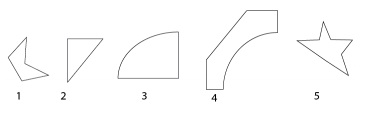A    1,4,2

B    1,2,3

C    1,4,5

D    2,3,4

Explanation:

If we combine images of 2,3,4 , we get a square.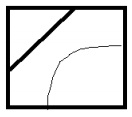So, the answer would be option d)2,3,4

Question 57. Four of the following five are alike in a certain way and so form a group. Which is the one that does not belong to that group? U, R, Y, S,W

A    Y

B    S

C    W

D    R

Explanation:

In the group, U, R, Y, S, W,

All letters U,Y, S & W are in odd positions as per alphabetical order whereas R is in even position. Hence, R is right choice.

Question 58. In a certain code language, if CRACK is coded as TEZME, then how is AMUCK coded in that language?

A    OCTEM

B    COTEM

C    COTME

D    OCTME

Question 59. In this question, a passage is given followed by a statement. Read the passage carefully and judge the statement based on the given passage. When Shah Jahan eternalised his love for Mumtaz, he must have believed that the river would be flowing forever. There was no solid ground on which the foundation could be built. So they used a well foundation, an engineering practice using deep wells to lower the water table and filling them with rocks and mortar. Finally they were capped with wooden, boxlike structures on which the mausoleum was constructed. The Yamuna is an integral part of the Taj’s design and there was no anticipation that it would dry or become narrow. But the river has narrowed and it is polluted. The wooden foundations are likely to become brittle and might disintegrate because, as Venice, built on a wooden foundation, shows, longevity of wood increases when it is submerged in water. When the wood is not exposed to oxygen there are no microorganisms to promote decay. A dry Yamuna could promote disintegration of the wooden foundations, the corrosive effect of the polluted water adding to the decay.

Statement: To retain the structural integrity of the Taj Mahal, the river Yamuna must be restored and revived.

Choose the appropriate one from the following options

A -The statement is definitely true.

B -The statement is probably true.

C -The statement is cannot be det ermined.

D -The statement is definitely false.

A    D

B    A

C   C

D   B

Question 60. Choose the correct alternative which will complete the image pattern of the given image.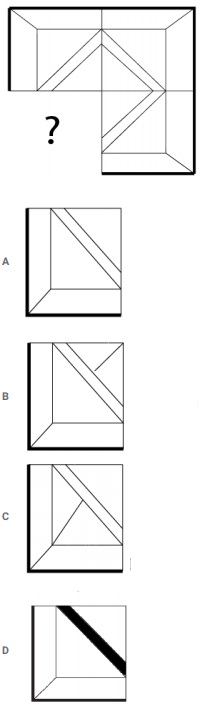Explanation: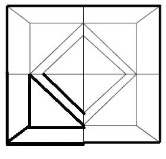So, the answer would be option a.

RPF SI Solved Previous Papers

Question 61. In this question, three statements are given followed by three conclusions. You have to assume everything in the statement to be true, then consider the given conclusions together and decide which of them logically follows beyond a reasonable doubt from the information given in the statements.

Statements: Some workers are teachers. Some teachers are lecturers. All lecturers are professionals.

Conclusions:

i) Some profe ssionals are teachers.

ii) Some professionals are workers.

iii) All lecturers are teachers.

A    Only i) and iii) follow

B    Only iii) follows

C    Only ii) and iii) follow

D    Only i)

Question 62. In this question, three statements showing relationship have been given, which are followed by three conclusions i, ii and iii. Assuming that the given statements are true, find out which conclusion(s) is/are definitely true.

Statements: C ≤ U < E; C = O > M ≥ T; M = A > L

Conclusions:

i) E > L

ii) E > T

iii) U > O

A    Only ii and iii follow

B    Only iii follows

C    Only i and ii follow

D    All follow

Question 63. Four of the following five are alike in a certain way and so form a group. Which is the one that does not belong to that group?

JNR, DHL, CGK, EIM, F KP

A   FKP

B   CGK

C   JNR

D   DHL

Explanation:

In given terms, In each term, 1st & 2nd letters have 3letters between them. Similarly, 2nd and 3rd letters also have 3letters between them.

Such pattern is not observed in FKP. Hence, Option A is right choice.

Question 64. Four of the following five are alike in a certain way and so form a group. Which is the one that does not belong to that group?

Sorrow, Sad, Regret, A wful, Comic

A    Awful

B    Sorrow

C    Regret

D    Comic

Explanation:

In given list of words, All words except Comic are synonyms. Hence, Comic doesn't belong to group.

Question 65. How many squares are there in the given figure?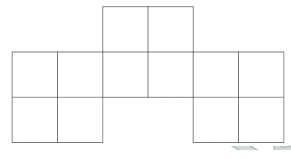A    17

B    9

C    15

D    12

Explanation:

There are 3 big squares , and inside each of them there are 4 squares.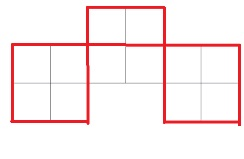So, the answer would be option c)15.

## RRB Railway Protection Force (RPF) Constable Exam

Question 66. Read the given information carefully and answer the questions that follow.

7 professionals A, B, C, D, E, F and G live in a building which has only 2 floor s numbered I and II. Out of the 2 floors one floor has 3 houses and the other floor has 4 houses.

i) G lives in the floor which has 3 houses.

ii) The floor in which D lives is not the sam e floor in which G and B live.

iii) C and A live in different floors.

iv) There is one house between th e houses in which F and A live.

Which of the following statements is false with respect to the clues given in the question?

A    C, G, and B live in floor II

B    G and B are neighbours

C    F and A are not neighbours

D    D and E live in the same floor

Question 67. In this question, relationship between different elements is shown in the statement. This statement is followed by two conclusions:

Statements: M ≤ N < O ≥ P < Q

Conclusion:

i) M < P

ii) O < Q

Choose the appropriate one from the following options

(A) Only conclusion i follows

(B) Only conclusion ii follows

(C) Either i or ii follows

(D) Neither i nor ii follow s

(E) Both i and ii follow.

A    C

B    B

C    D

D    A

Question 68. In a certain code language, if GIFTED is coded as TRUGVW, then how is LUCID coded in that language?

A    MWDKF

B    OFRXW

C    OFXRW

D    MVDJE

Question 69. Find the next number in the series.

33, 35, 38, 43, 50, ?

A    63

B    62

C    64

D    61

Explanation:

Difference between n and n-1 term is a prime number. Hence, next prime number that gets added to 50 would be 11.

Question 70. If a mirror is placed on the shaded line, then which of the following options is the right image of the given figure?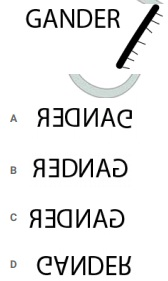Question 71. Find the next number in the series.

11, 16, 32, 37, 74, ?

A    75

B    79

C    81

D    77

Question 72. In this question, a passage is given followed by a statement. Read the passage carefully and judge the statement based on the given passage. When Shah Jahan eternalised his love for Mumtaz, he must have believed that the river would be flowing forever. There was no solid ground on which the foundation could be built. So they used a well foundation, an engineering practice using deep wells to lower the water table and filling them with rocks and mortar. Finally they were capped with wooden, boxlike structures on which the mausoleum was constructed. The Yamuna is an integral part of the Taj’s design and there was no anticipation that it would dry or become narrow. But the river has narrowed and it is polluted. The wooden foundations are likely to become brittle and might disintegrate because, as Venice, built on a wooden foundation, shows, longevity of wood increases when it is submerged in water. When the wood is not exposed to oxygen there are no microorganisms to promote decay. A dry Yamuna could promote disintegration of the wooden foundations, the corrosive effect of the polluted water adding to the decay.

Statement: Taj Mahal was built on a strong solid ground on the bank of river Yamuna.

Choose the appropriate one from the following options

A -The statement is definitely true.

B -The statement is probably true.

C -The statement is cannot be det ermined.

D -The statement is definitely false.

A    C

B    D

C    A

D    B

Question 73. Replace the question mark with an option that follows the same logic applied in the first pair Eating:Restaurant::Exercise: ??

A    Health

B    Sports

C    Sweat

D    Gym

Explanation:

Activity we do in a restaurant would be Eating. Activity like Excersise would be done in "Gym" which is Option D. Hence, It's right choice.

Question 74. How many Right angled triangles can be formed from the given figure?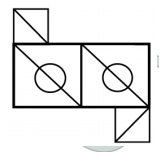A    5

B    10

C    6

D    8

Question 75. Each face of the dice is printed with 6 letters A,B,C,D,E and F as shown in the given figure, What letter is printed on the face opposite to letter A?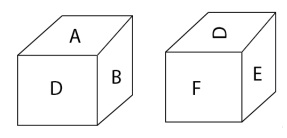A    F

B    E

C    C

D    D

Question 76. Choose the correct water image of the given question from the given options.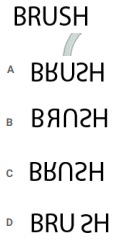Question 77. A is the son-in-law of B whose son is D. D is the brother of C who is the only daughter of E. How is C related to A?

A    Cousin

B    Husband

C    Wife

D    Sister-in-law

Question 78. Replace the question mark with an option that follows the same logic applied in the first pair

1125:18::1356: ??

A    40

B    10

C    20

D    30

Explanation:

In the given question, 1125:18::1356: ??

We can see that Sum of digits of 1125 will be 9. When it's multiplied by 2, we get 18.

Similarly, Sum of number 1356 will be 15 and when it's multiplied by 2, we get 30.

Question 79. Read the given information carefully and answer the questions that follow.

7 professionals A, B, C, D, E, F and G live in a building which has only 2 floor s numbered I and II. Out of the 2 floors one floor has 3 houses and the other floor has 4 houses.

i) G lives in the floor which has 3 houses.

ii) The floor in which D lives is not the sam e floor in which G and B live.

iii) C and A live in different floors.

iv) There is one house between th e houses in which F and A live.

Which of the following is the odd one out with respect to the clues given in the question?

A   G

B   D

C   F

D   E

Question 80. Find the next number in the series.

12, 25, 52, 107, 218, ?

A    441

B    440

C    421

D    431

Explanation:

In the Given series 12, 25, 52, 107, 218, ?

We can observe the pattern , ,  , ,

Similarly, next term would be  = 441

Question 81. Read the given information carefully and answer the questions that follow.

7 professionals A, B, C, D, E, F and G live in a building which has only 2 floor s numbered I and II. Out of the 2 floors one floor has 3 houses and the other floor has 4 houses.

i) G lives in the floor which has 3 houses.

ii) The floor in which D lives is not the sam e floor in which G and B live.

iii) C and A live in different floors.

iv) There is one house between th e houses in which F and A live.

Which of the following options has a group of people who live in the same floor?

A    C, B & D

B    C, G & E

C    F, A & E

D    D, E & G

Question 82. Pointing to a little girl, a lady said, "She is the daughter of sister of brother of my husband's sister." How is the lady related to the little girl?

A    Cousin

B   Aunt

C  Grandmother

D  Mother

Question 83. Find the next number in the series.

43, 44, 48, 57, 73, ?

A    97

B    100

C    98

D    99

Explanation:

In the given series, 43, 44, 48, 57, 73, ?, There's another series formed by difference between two terms i.e.., 1,4,9,16,..

Next term in series would be 25 and it would be added to 73 to get next number in main series. therefore, next number is 98.

Question 84. In this question, a statement is given followed by two conclusions numbered i and ii. You have to assume everything in the statement to be true, then consider the two conclusions together and decide which of them logically follows beyond a reasonable doubt from the information given in the statement.

Statement: It rains every Monday. It rains today.

Conclusion:

i) It must be a rainy season.

ii) Today is Monday

Choose the appropriate one from the following options

(A) Only conclusion i follows

(B) Only conclusion ii follows

(C) Either i or ii follows

(D) Neither i nor ii follow s

(E) Both i and ii follow.

A    A

B    B

C    C

D    D

Explanation:

Statement: It rains every Monday. It rains today.

Conclusion:

i) It must be a rainy season. - Although it rains every Monday, it does not justify the month to be rainy.

ii) Today is Monday - It rains every Monday , but there is possibility that it might rain other day also.

None of the conclusions follow.

So, the answer would be option d)D

A+B means A is the mother of B

A-B means A is the father of B

A*B means A is the sister of B

A/B means A is the brother of B

In the equation W+X/Y-Z, how is X's wife related to Z?

A    Aunt

B    Cousin

C    Grandmother

D    Mother

## << Go Back to Main Page

IMPORTANT: RRB EXAM PORTAL is NOT associated with Railway Recruitment Board(RRB) or Indian Railways, For RRB official website visit - www.rrcb.gov.in